Скачать презентацию כנס האיכות הרביעי בגליל איכות הלכה

5c862c9e96a477cf4b72bba94b535f4a.ppt

• Количество слайдов: 28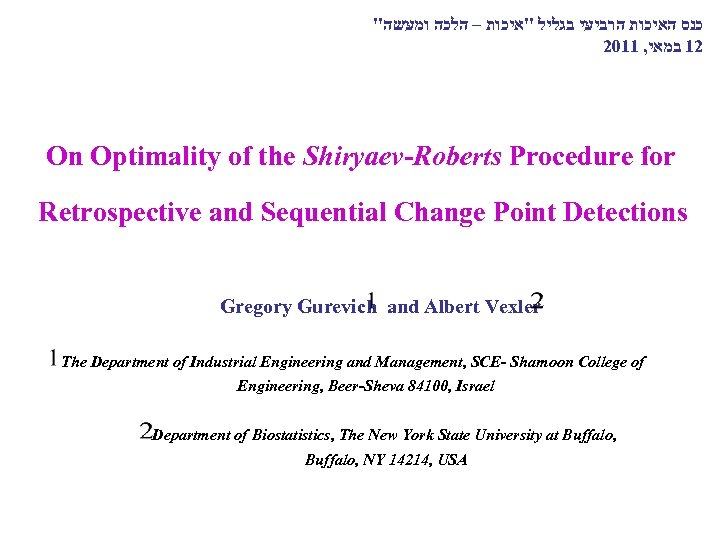" כנס האיכות הרביעי בגליל "איכות – הלכה ומעשה 2011 , 21 במאי On Optimality of the Shiryaev-Roberts Procedure for Retrospective and Sequential Change Point Detections Gregory Gurevich and Albert Vexler The Department of Industrial Engineering and Management, SCE- Shamoon College of Engineering, Beer-Sheva 84100, Israel Department of Biostatistics, The New York State University at Buffalo, NY 14214, USA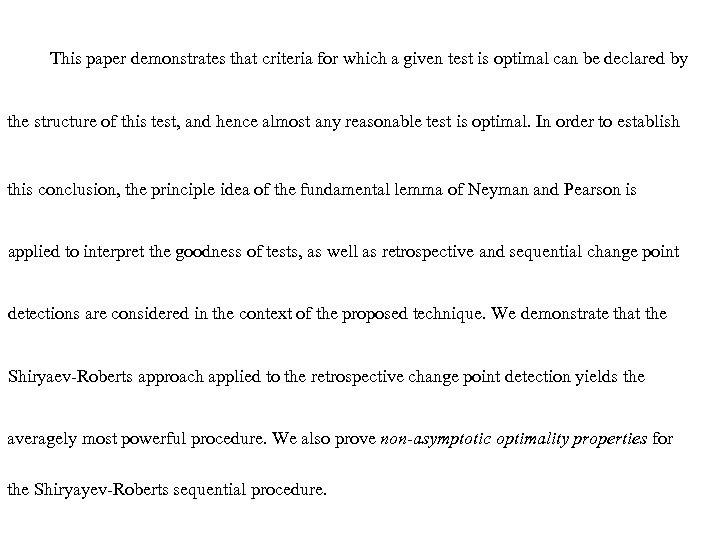This paper demonstrates that criteria for which a given test is optimal can be declared by the structure of this test, and hence almost any reasonable test is optimal. In order to establish this conclusion, the principle idea of the fundamental lemma of Neyman and Pearson is applied to interpret the goodness of tests, as well as retrospective and sequential change point detections are considered in the context of the proposed technique. We demonstrate that the Shiryaev-Roberts approach applied to the retrospective change point detection yields the averagely most powerful procedure. We also prove non-asymptotic optimality properties for the Shiryayev-Roberts sequential procedure.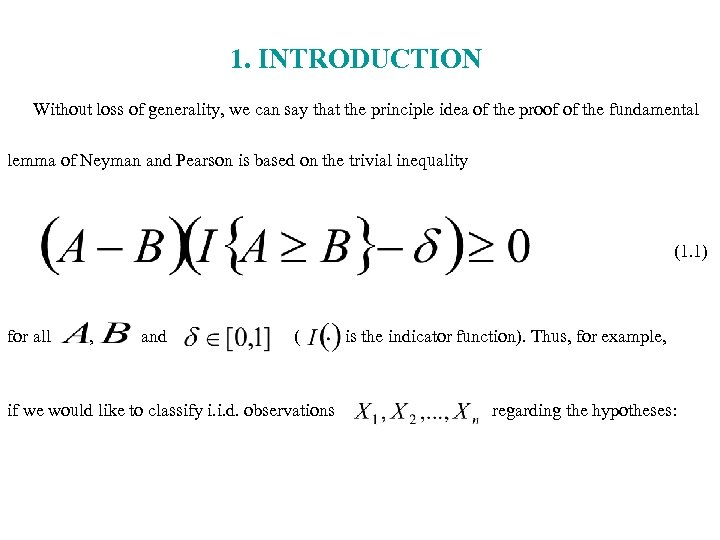1. INTRODUCTION Without loss of generality, we can say that the principle idea of the proof of the fundamental lemma of Neyman and Pearson is based on the trivial inequality (1. 1) for all , and ( if we would like to classify i. i. d. observations is the indicator function). Thus, for example, regarding the hypotheses: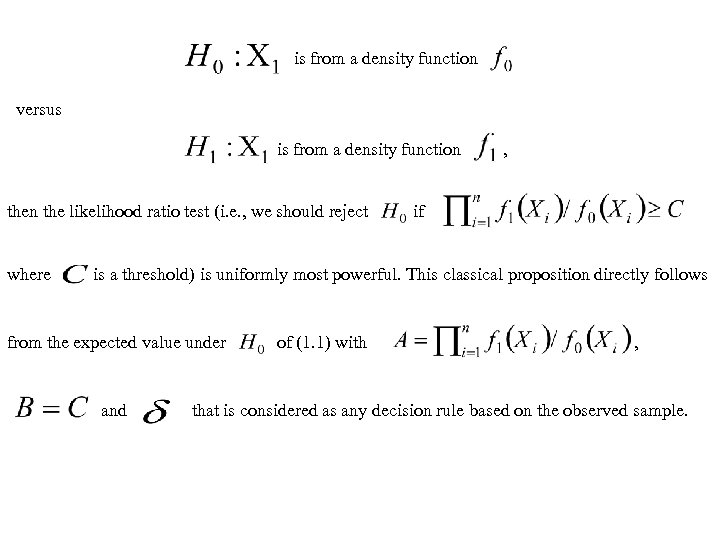is from a density function versus is from a density function the likelihood ratio test (i. e. , we should reject where , if is a threshold) is uniformly most powerful. This classical proposition directly follows from the expected value under and of (1. 1) with , that is considered as any decision rule based on the observed sample.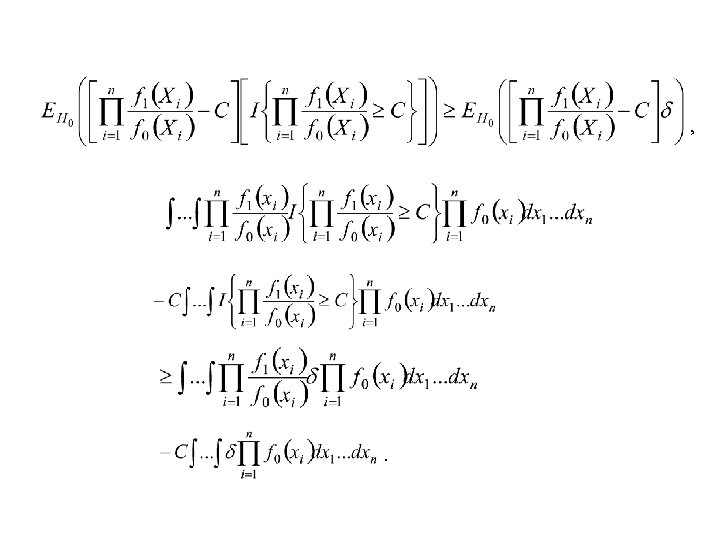, .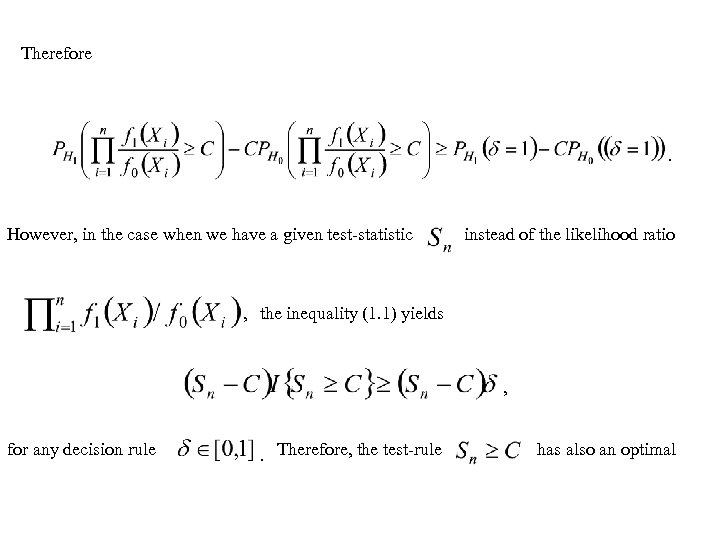Therefore . However, in the case when we have a given test-statistic instead of the likelihood ratio , the inequality (1. 1) yields , for any decision rule . Therefore, the test-rule has also an optimal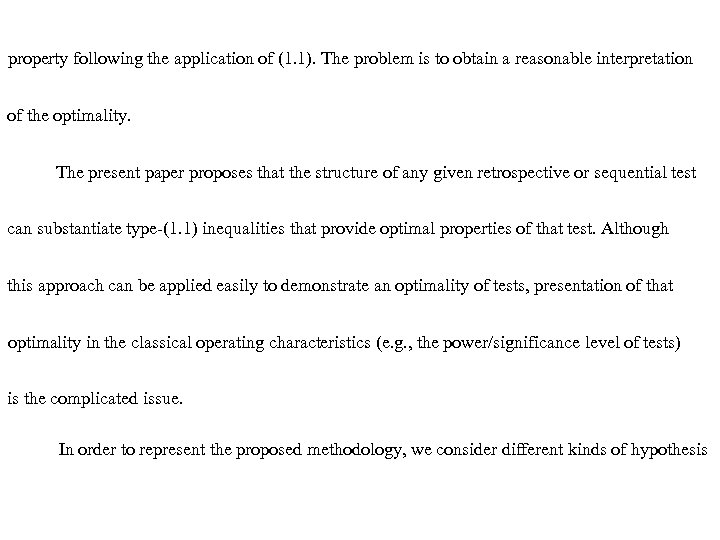property following the application of (1. 1). The problem is to obtain a reasonable interpretation of the optimality. The present paper proposes that the structure of any given retrospective or sequential test can substantiate type-(1. 1) inequalities that provide optimal properties of that test. Although this approach can be applied easily to demonstrate an optimality of tests, presentation of that optimality in the classical operating characteristics (e. g. , the power/significance level of tests) is the complicated issue. In order to represent the proposed methodology, we consider different kinds of hypothesis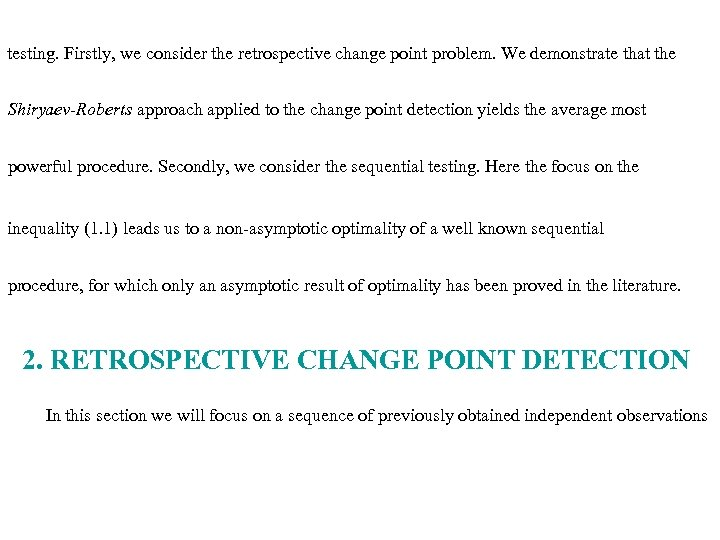testing. Firstly, we consider the retrospective change point problem. We demonstrate that the Shiryaev-Roberts approach applied to the change point detection yields the average most powerful procedure. Secondly, we consider the sequential testing. Here the focus on the inequality (1. 1) leads us to a non-asymptotic optimality of a well known sequential procedure, for which only an asymptotic result of optimality has been proved in the literature. 2. RETROSPECTIVE CHANGE POINT DETECTION In this section we will focus on a sequence of previously obtained independent observations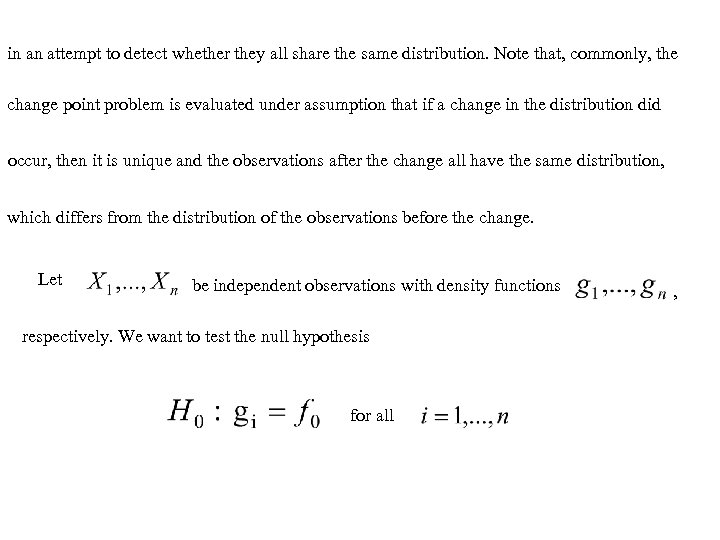in an attempt to detect whether they all share the same distribution. Note that, commonly, the change point problem is evaluated under assumption that if a change in the distribution did occur, then it is unique and the observations after the change all have the same distribution, which differs from the distribution of the observations before the change. Let be independent observations with density functions respectively. We want to test the null hypothesis for all ,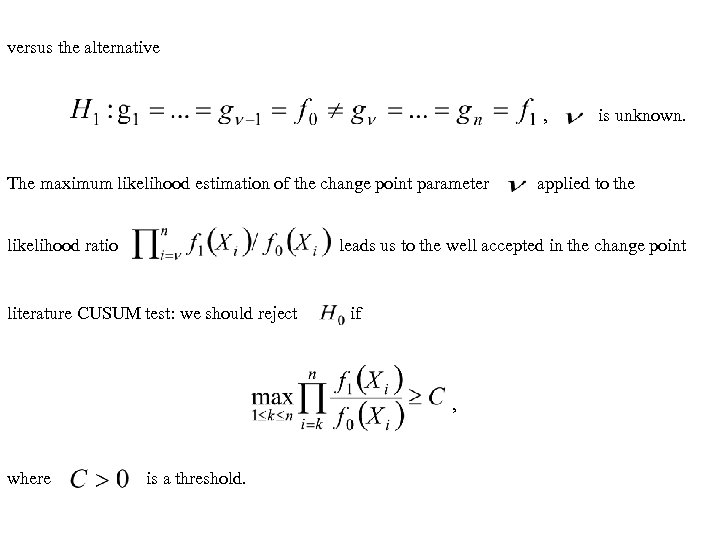versus the alternative , The maximum likelihood estimation of the change point parameter likelihood ratio applied to the leads us to the well accepted in the change point literature CUSUM test: we should reject if , where is unknown. is a threshold.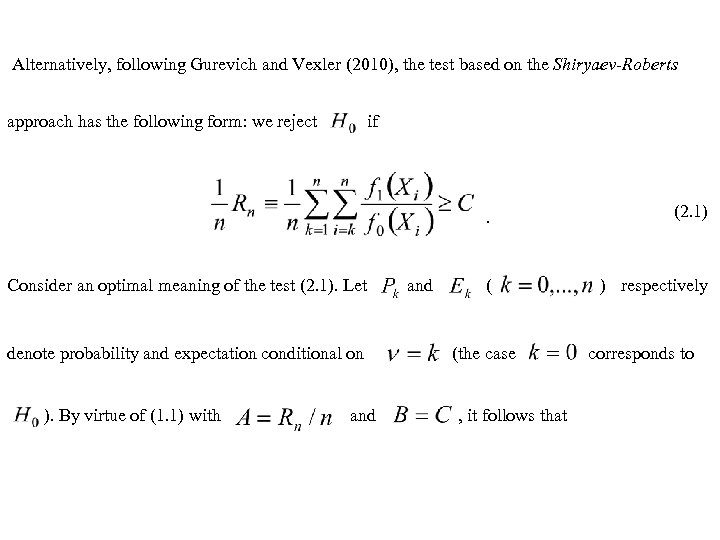Alternatively, following Gurevich and Vexler (2010), the test based on the Shiryaev-Roberts approach has the following form: we reject if . Consider an optimal meaning of the test (2. 1). Let denote probability and expectation conditional on ). By virtue of (1. 1) with and (2. 1) ( ) respectively (the case , it follows that corresponds to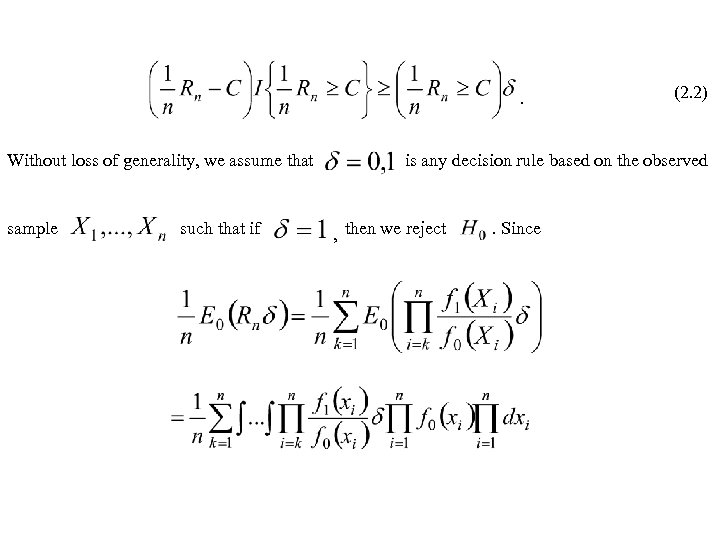. Without loss of generality, we assume that sample such that if (2. 2) is any decision rule based on the observed , then we reject . Since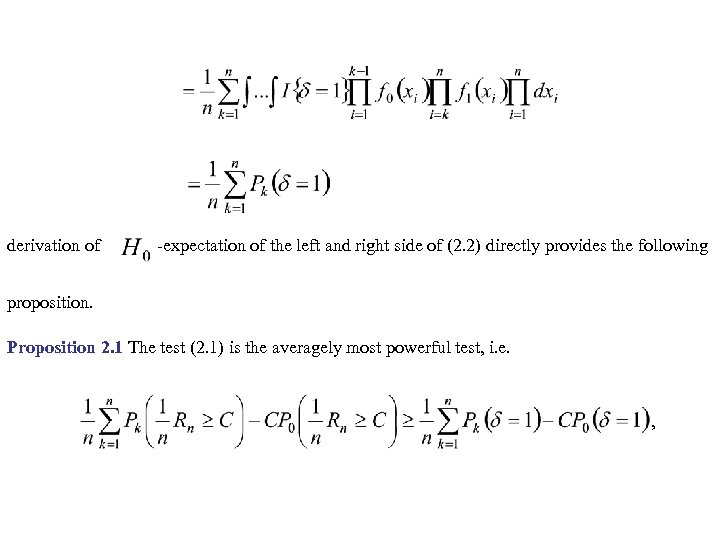derivation of -expectation of the left and right side of (2. 2) directly provides the following proposition. Proposition 2. 1 The test (2. 1) is the averagely most powerful test, i. e. ,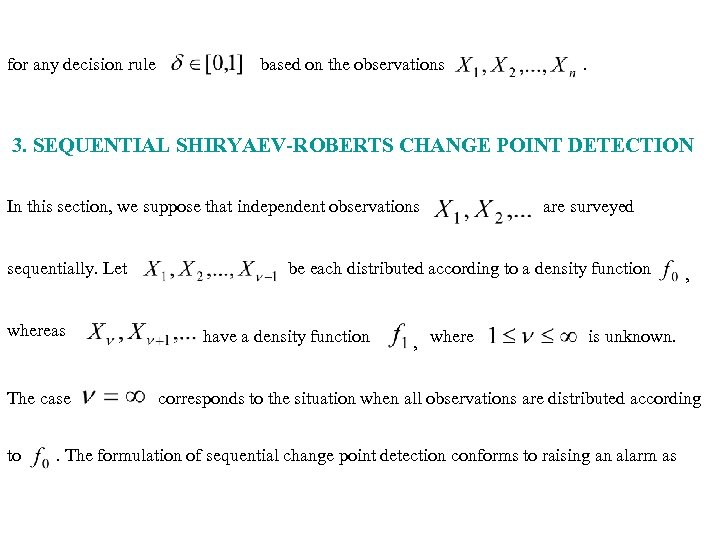for any decision rule based on the observations . 3. SEQUENTIAL SHIRYAEV-ROBERTS CHANGE POINT DETECTION In this section, we suppose that independent observations sequentially. Let whereas The case to are surveyed be each distributed according to a density function have a density function , where , is unknown. corresponds to the situation when all observations are distributed according . The formulation of sequential change point detection conforms to raising an alarm as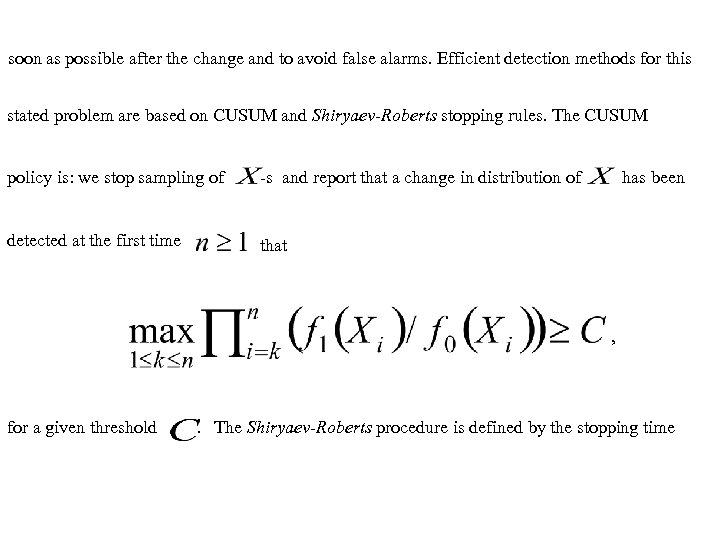soon as possible after the change and to avoid false alarms. Efficient detection methods for this stated problem are based on CUSUM and Shiryaev-Roberts stopping rules. The CUSUM policy is: we stop sampling of -s and report that a change in distribution of detected at the first time has been that , for a given threshold . The Shiryaev-Roberts procedure is defined by the stopping time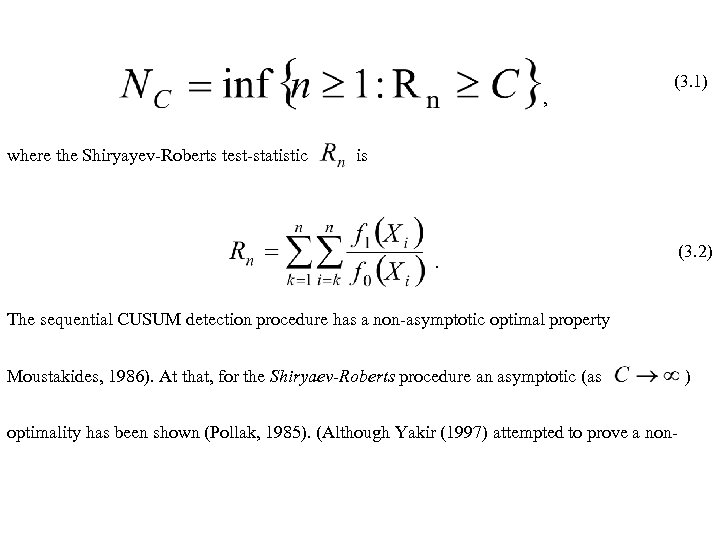, where the Shiryayev-Roberts test-statistic (3. 1) is . (3. 2) The sequential CUSUM detection procedure has a non-asymptotic optimal property Moustakides, 1986). At that, for the Shiryaev-Roberts procedure an asymptotic (as optimality has been shown (Pollak, 1985). (Although Yakir (1997) attempted to prove a non- )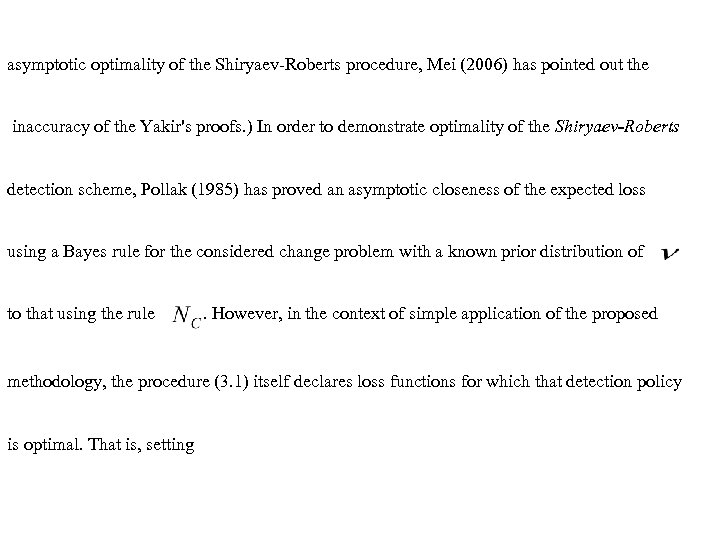asymptotic optimality of the Shiryaev-Roberts procedure, Mei (2006) has pointed out the inaccuracy of the Yakir's proofs. ) In order to demonstrate optimality of the Shiryaev-Roberts detection scheme, Pollak (1985) has proved an asymptotic closeness of the expected loss using a Bayes rule for the considered change problem with a known prior distribution of to that using the rule . However, in the context of simple application of the proposed methodology, the procedure (3. 1) itself declares loss functions for which that detection policy is optimal. That is, setting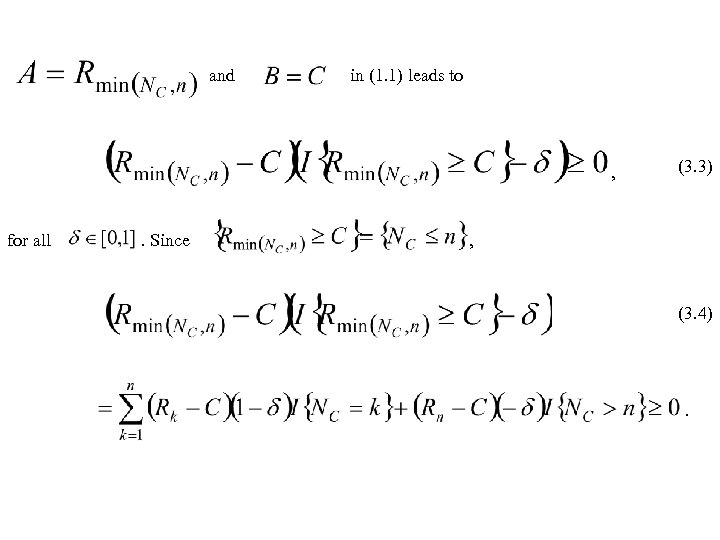and in (1. 1) leads to , for all . Since (3. 3) , (3. 4) .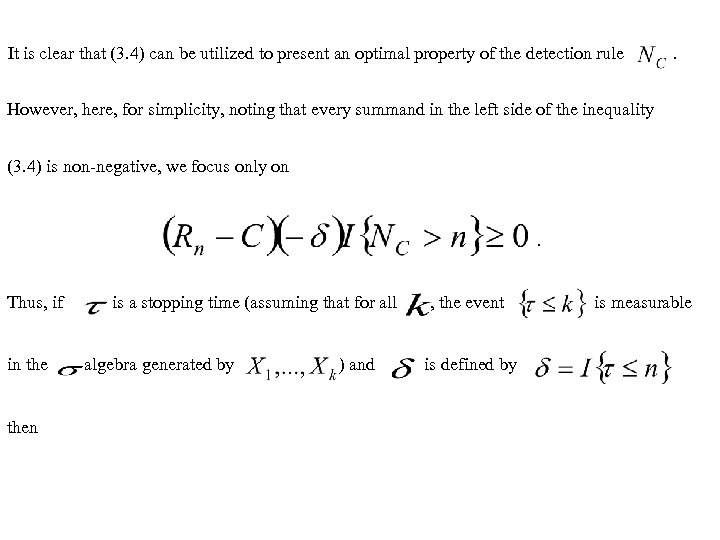It is clear that (3. 4) can be utilized to present an optimal property of the detection rule . However, here, for simplicity, noting that every summand in the left side of the inequality (3. 4) is non-negative, we focus only on . Thus, if in then is a stopping time (assuming that for all -algebra generated by ) and , the event is defined by is measurable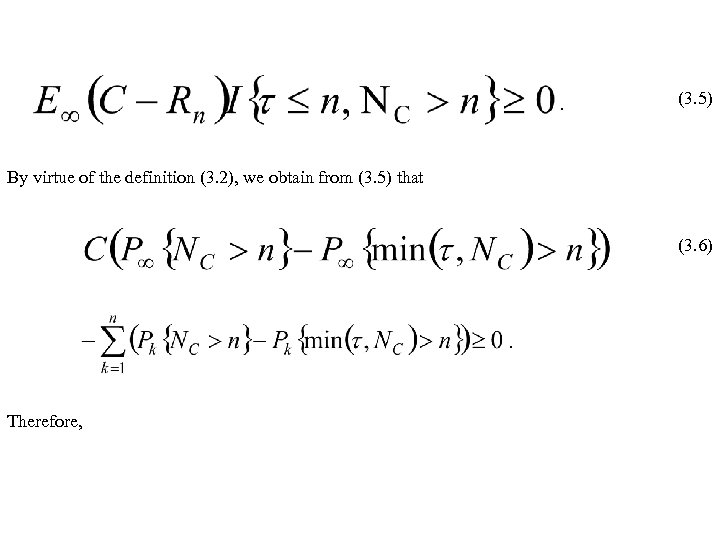. (3. 5) By virtue of the definition (3. 2), we obtain from (3. 5) that (3. 6) . Therefore,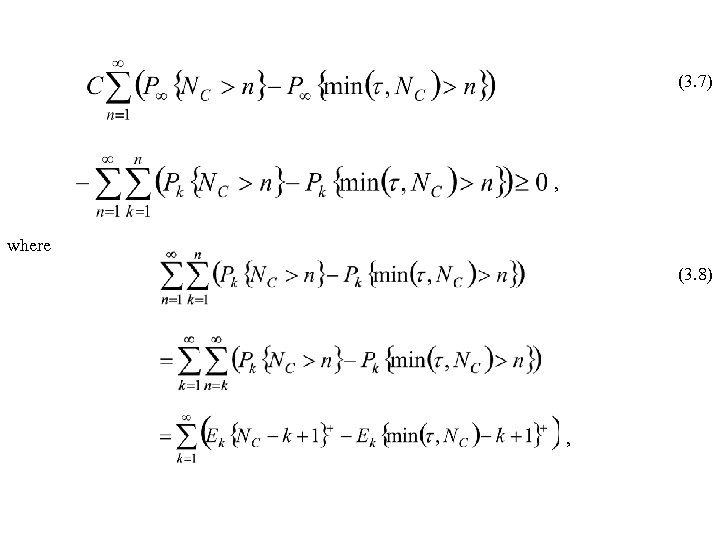(3. 7) , where (3. 8) ,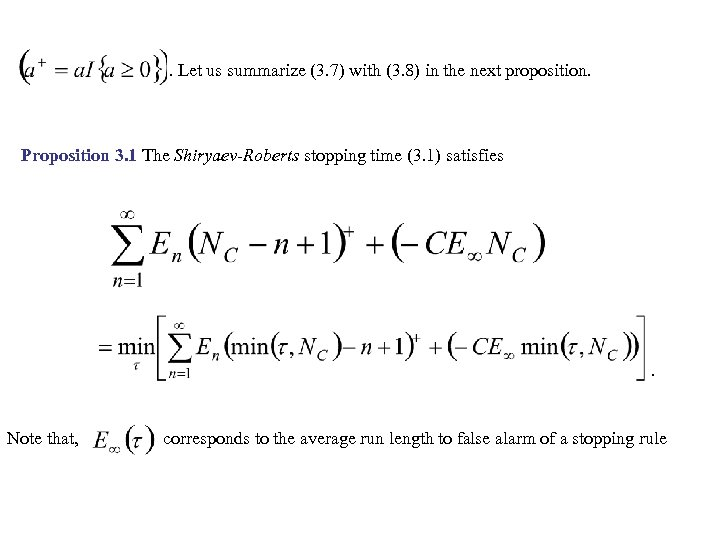. Let us summarize (3. 7) with (3. 8) in the next proposition. Proposition 3. 1 The Shiryaev-Roberts stopping time (3. 1) satisfies . Note that, corresponds to the average run length to false alarm of a stopping rule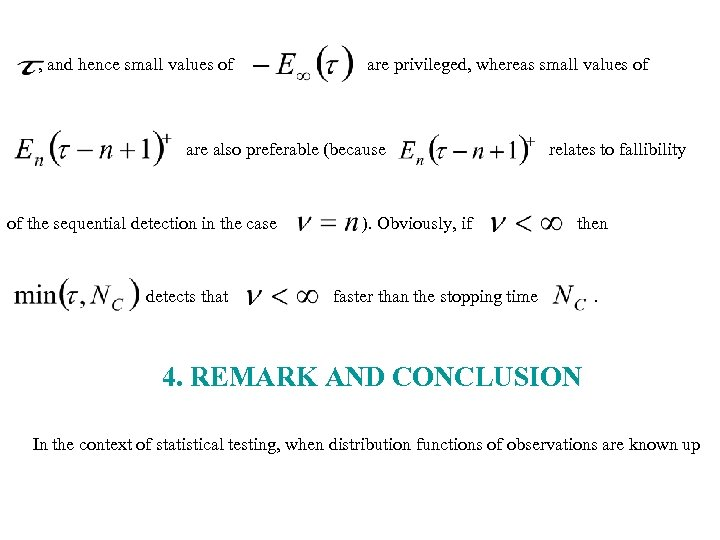, and hence small values of are privileged, whereas small values of are also preferable (because of the sequential detection in the case detects that ). Obviously, if relates to fallibility then faster than the stopping time . 4. REMARK AND CONCLUSION In the context of statistical testing, when distribution functions of observations are known up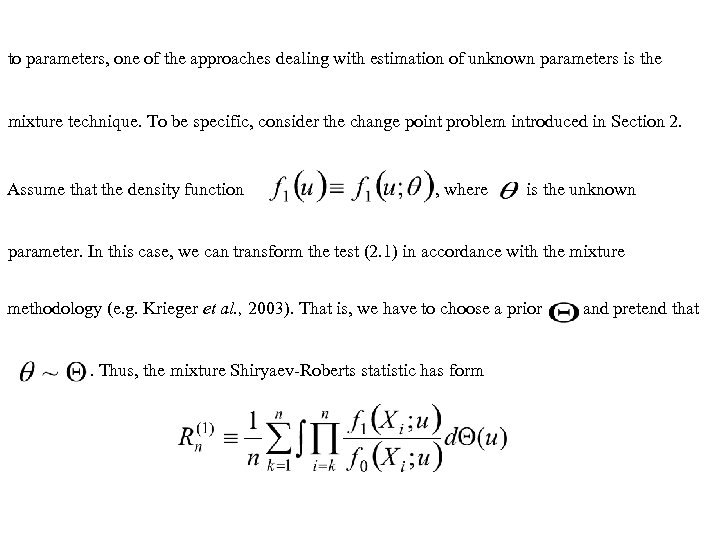to parameters, one of the approaches dealing with estimation of unknown parameters is the mixture technique. To be specific, consider the change point problem introduced in Section 2. Assume that the density function , where is the unknown parameter. In this case, we can transform the test (2. 1) in accordance with the mixture methodology (e. g. Krieger et al. , 2003). That is, we have to choose a prior. Thus, the mixture Shiryaev-Roberts statistic has form and pretend that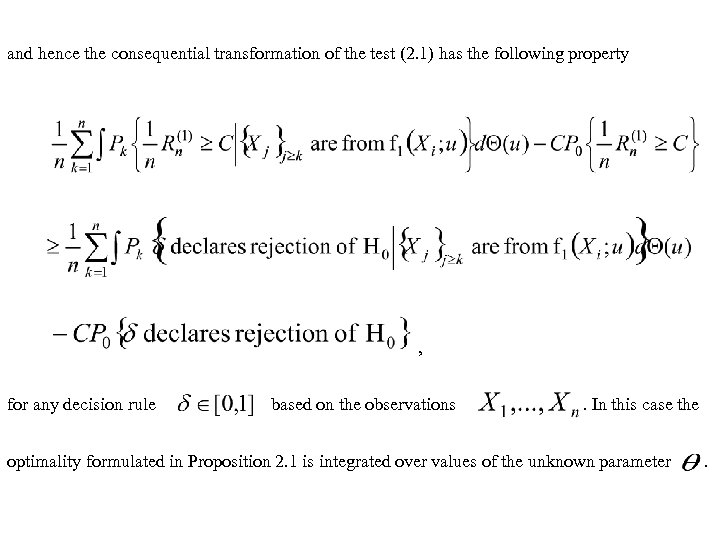and hence the consequential transformation of the test (2. 1) has the following property , for any decision rule based on the observations . In this case the optimality formulated in Proposition 2. 1 is integrated over values of the unknown parameter .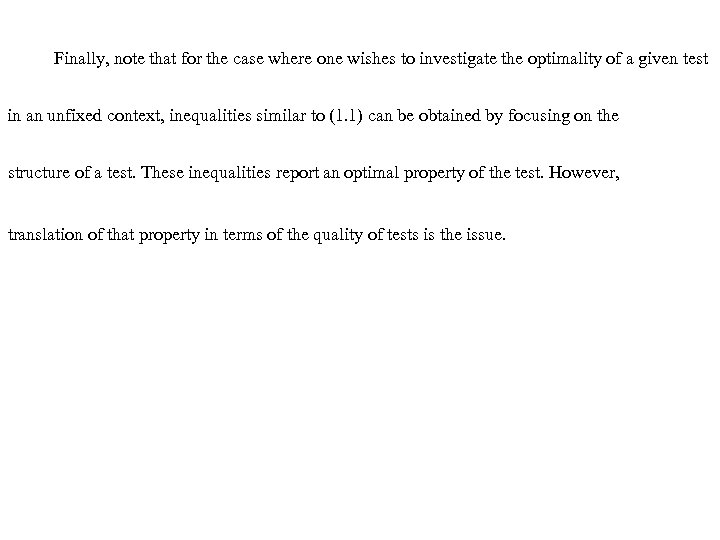Finally, note that for the case where one wishes to investigate the optimality of a given test in an unfixed context, inequalities similar to (1. 1) can be obtained by focusing on the structure of a test. These inequalities report an optimal property of the test. However, translation of that property in terms of the quality of tests is the issue.Questions?Thank you!